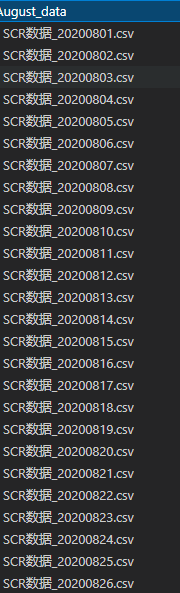出租广告位,需要合作请联系站长

+关注

2020-10(6)

2020-11(13)

## Python处理(加载、合并)多个csv文件

数据集介绍：本数据集是某化工系统的数据，一共有很多个月的，我这里就拿一个月的数据集，August_data（八月的数据集)，一共有31个csv文件。### 方法一  for循环遍历+os.listdir(directory_path)+[ for file in tqdm] + os.path.join(path,file)

``import pandas as pdimport numpy as np from tqdm import tqdmimport os def get_data(path):    df_list = []    for file in tqdm(os.listdir(path)):##进度条        file_path = os.path.join(path, file)        df = pd.read_csv(file_path)        df_list.append(df)    df = pd.concat(df_list)    return df cPath = '.\August_data'# cPath = 'F:/BaiduNetdiskDownload/宁东电厂数据及分析要求/宁东脱销系统优化-上海交大/SCR数据-2020-1/8月数据' #F:/BaiduNetdiskDownload/宁东电厂数据及分析要求/宁东脱销系统优化-上海交大/SCR数据-2020-1/8月数据# uPath = str(cPath)#uPath = unicode(cPath,'utf-8')# dirs = os.listdir(TEST_PATH)# print(dirs)test_df = get_data(cPath)print(test_df.head())# test_df.to_csv(path_or_buf="test.csv",index=False)#保存为CSV文件``

### 方法二   glob方法

`` #!/usr/bin/env python# coding=utf-8 import globimport timeimport csvimport pandas as pdfrom tqdm import tqdm# a new file #open all the CSV file#遍历文件夹下所有csv文件TEST_PATH = '.\August_data' csv_list = glob.glob(f'{TEST_PATH}\*.csv')print('共有%s个CSV文件'% len(csv_list))# print (csv_list) def get_data():    df_list = []    for csv_file in csv_list:        df = pd.read_csv(csv_file)        df_list.append(df)    df = pd.concat(df_list)    print("Loading Oer")    return dfget_data()``

### 参考

Python --读取多个CSV文件特定行写入到新文件

glob模块使用教程​​​​​​​

glob模式匹配的规则​​​​​​​N​​​​​​​z

pandas 之unique()函数与nunique()函数区别

两个办法都挺好的，浅层原理都是，得到文件夹下的各个文件的名字，然后利用该名字做遍历去加载csv。

# 如果目录名字为中文 需要转码处理：uPath = unicode(cPath,'utf-8')，但python2中的unicode()函数在python3中会报错：Python3没有unicode()这个函数，换成了 str()函数，但如果是csv本身数据不合规的处理起来还是麻烦，本身这套打法没问题。

4 0

pdf(new) 更多>